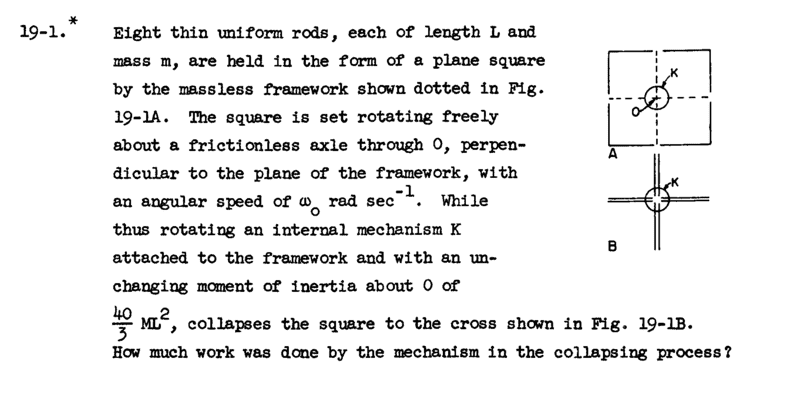# Feynman Exercises 19-1: Metal rod framework being pulled in while spinning

aa_o

## Homework Statement## Homework Equations## The Attempt at a Solution

The moment of inertia before collapse is for each rod:
BEFORE COLLAPSE:[/B]
Ib = ∫(L2 + x2) dm = m/L ∫(L2 + x2) dx = 4/3 * m * L2
We have 8 of these plus the inertia of the mechanism, giving a total I,
It = 8 * Ib + Ik = (32/3 * m * + 40/3 * M) * L^2
The energy is thus:
Tb = 1/2 * It* ωo2
AFTER COLLAPSE:
Ia = ∫(x2) dm = m/L ∫(x2) dx = 1/3 * m * L2
And the mechanism is the same:
So we have total of:
It_a = 8 * Ia + Ik = (8/3 * m * + 40/3 * M) * L2
The energy is now:
Ta = 1/2 * It_a * ωo2
And energy difference must be:
Ta - Tb = 1/2 * ωo2 * m * (8 / 3 - 32/3) * L2 = -ωo2 * m * 4 * L2

But the solution stated is: ωo2 * M * 6 (note the difference in mass symbol)
What am i doing wrong?

Last edited:

## Answers and Replies

Homework Helper
Gold Member
2021 Award
Why is the angular speed unchanged through the collapse?

aa_o
Why is the angular speed unchanged through the collapse?
Because the moment of inertia of the mechanism is unchanged. Since I = L / ω (L is the angular momentum here!).

But maybe I've been to quick in that assumption. All I know is that I is constant for the mechanism - not that L or ω is constant. Am I on the right track?

Homework Helper
Gold Member
2021 Award
Because the moment of inertia of the mechanism is unchanged. Since I = L / ω (L is the angular momentum here!).

But maybe I've been to quick in that assumption. All I know is that I is constant for the mechanism - not that L or ω is constant. Am I on the right track?
Sure the moment of inertia of the mechanism is unchanged, but what about the framework? Is that also unchanged? Your expressions for It and It_a are not the same. In fact It_a < It which means that the contraption will speed up much like a spinning skater when she pulls her arms in.,

Last edited:
aa_o
Sure the moment of inertia of the mechanism is unchanged, but what about the framework? Is that also unchanged? Your expressions for It and It_a are not the same. In fact It_a < It which means that the contraption will speed up much like a spinning skater when she pulls her arms in.,
Ahh, okay. There's conservation of angular momentum. I'll work it out and return with what i got.

Homework Helper
Gold Member
2021 Award
Ahh, okay. There's conservation of angular momentum. I'll work it out and return with what i got.
That's an excellent way to proceed. Note: To get the answer you think is correct, you must set ##m=M##.

aa_o
That's an excellent way to proceed. Note: To get the answer you think is correct, you must set ##m=M##.
Ahh yes! Thanks a lot. Quick last question: Do you know if the different symbol for mass is a mistake in the problem? Or am i missing some connection?

To anyone else stuck here:

Just note that angular momemtum is conserved, meaning: I_t * ω_0 = I_t_a * ω_a (ω_a is angular velocity after collapse). After this, it's just algebra.